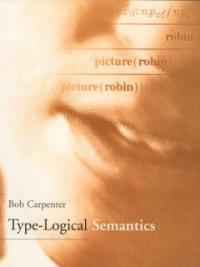> Detail View

# Detail View## Type-logical semantics

Material type
단행본
Personal Author
Carpenter, Bob.
Title Statement
Type-logical semantics / Bob Carpenter.
Publication, Distribution, etc
Cambridge, Mass. :   MIT Press ,   1998.
Physical Medium
xxi, 575 p. ; 23 cm.
Series Statement
Language, speech, and communication
ISBN
0262032481 (cloth : alk. paper) 0262531496 (pbk. : alk. paper)
General Note
Bibliography, Etc. Note
Includes bibliographical references (p. -568) and index.
Semantics. Semantics (Philosophy) Language and logic. Grammar, Comparative and general. Computational linguistics. Logic.
 000 01041camuu2200325 a 4500 001 000045185468 005 20050826095122 008 970127s1997 mau b 001 0 eng 010 ▼a 97000863 020 ▼a 0262032481 (cloth : alk. paper) 020 ▼a 0262531496 (pbk. : alk. paper) 040 ▼a DLC ▼c DLC ▼d DLC ▼d 211009 050 0 0 ▼a P325 ▼b .C324 1997 082 0 0 ▼a 401/.43 ▼2 21 090 ▼a 401.43 ▼b C295t 100 1 ▼a Carpenter, Bob. 245 1 0 ▼a Type-logical semantics / ▼c Bob Carpenter. 260 ▼a Cambridge, Mass. : ▼b MIT Press , ▼c 1998. 300 ▼a xxi, 575 p. ; ▼c 23 cm. 440 0 ▼a Language, speech, and communication 500 ▼a "A Bradford book." 504 ▼a Includes bibliographical references (p. -568) and index. 650 0 ▼a Semantics. 650 0 ▼a Semantics (Philosophy) 650 0 ▼a Language and logic. 650 0 ▼a Grammar, Comparative and general. 650 0 ▼a Computational linguistics. 650 0 ▼a Logic. 945 ▼a KINS

### Holdings Information

No. Location Call Number Accession No. Availability Due Date Make a Reservation Service
No. 1 Location Call Number 401.43 C295t Accession No. 121108820 Availability Available Due Date Make a Reservation Service

### Contents information

```
CONTENTS

Preface = xiii

Acknowledgements = xix

Chapter 1 : Introduction = 1

1.1 Truth and Reference = 1

1.2 Topics in Semantics = 10

1.3 Topics in Pragmatics = 22

1.4 Methodology = 30

Exercises = 35

Chapter 2 : Simply Typed λ-Calculus = 37

2.1 Simple Types = 39

2.2 λ-Terms = 41

2.3 Functional Models = 45

2.4 Proof Theory for Simply Typed λ-Calculus = 49

2.5 Combinators and Variable-Free Logic = 60

2.6 Products = 64

2.7 Sums = 69

Exercises = 72

Chapter 3 : Higher-Order Logic = 75

3.1 Higher-Order Syntax = 75

3.2 Higher-Order Models = 77

3.3 Quantifiers in Natural Language = 83

3.4 Negative Polarity Items = 94

3.5 Definite Descriptions = 96

3.6 Proof Theory for Higher-Order Logic = 101

Excrcise = 104

Chapter 4 : Applicative Categorial Grammar = 111

4.1 The Category System = 112

4.2 Semantic Domains = 114

4.3 Categorial Lexicons = 115

4.4 Phrase Structure = 116

4.5 A Categorial Lexicon = 119

4.7 Ambiguity, Vagueness, and Meaning Postulates = 126

Exercises = 135

Chapter 5 : The Lambek Calculus = 139

5.1 Lambek's Sequent Calculus = 140

5.2 The Natural-Deduction Lambek Calculus = 153

5.3 Products = 164

5.4 Categorial Grammar as Logic = 168

Exercises = 174

Chapter 6 : Coordination and Unbounded Dependencies = 177

6.1 Coordination = 177

6.2 Conjunctive and Disjunctive Categories = 187

6.3 Unbounded Dependency Constructions = 199

Exercises = 209

Chapter 7 : Quantifiers and Scope = 213

7.1 Quantifying In = 215

7.2 Cooper Storage = 216

7.3 Scoping Constructor = 220

7.4 Type Raising and Quantifier Coordination = 228

7.5 Embedded Quantifiers = 232

7.6 Quantifiers and Coordinate Structures = 241

7.7 Quantification and Negation = 244

7.8 Quantification and Definite Descriptions = 247

7.9 Possessives = 251

7.10 Indefinites = 254

7.11 Generics = 259

7.12 Comparatives = 263

7.13 Expletives and the Unit Type = 281

Exercises = 286

Chapter 8 : Plurals = 293

8.1 An Ontology of Groups = 293

8.2 A Plural Grammar = 297

8.3 Distributors and Collectors = 302

8.4 Coordination, Negation, and Argument Lowering = 307

8.6 Plural Quantification = 314

8.7 Partitives and Pseudopartitives = 318

8.8 Nonboolean Coordination = 322

8.9 Comitative Complements = 326

8.10 Mass Terms = 328

Exercises = 333

Chapter 9 Pronouns and Dependency = 339

9.1 Pronouns and Reflexives = 339

9.2 Pronouns and Agreement = 342

9.3 Pronouns as Variables = 344

9.4 A Quantificational Approach to Reflexives = 347

9.5 Plural Pronouns = 352

9.6 Reciprocals and Generalized Quantification = 354

9.7 Pied Piping = 364

9.8 Ellipsis and Sloppy Anaphora = 367

9.9 interrogatives = 368

Exercises = 373

Chapter 10 Modal Logic = 379

10.1 modes of Truth = 379

10.2 S5 : A Modal Logic of Necessity = 382

10.3 Indexicality = 390

10.4 General Modal Logics = 390

10.5 Strict Implication and Counterfactuals = 395

10.6 First-Order Tense Logics = 399

10.7 Tense Logic and Natural Language = 408

10.8 Temporal-Period Structures = 411

10.9 Higher-Order Modal Logic = 418

Exercises = 420

Chapter 11 Intensionality = 425

11.1 An Intemional Grammar = 425

11.2 Individual Concepts and Quantificational Definites = 450

11.3 Alternatives to Possible Worlds = 457

11.4 Lexical Relations = 465

Exercises = 471

Chapter 12 Tense and Aspect = 479

12.1 Reichenbach's Approach to Simple and Perfect Tenses = 479

12.2 Tense and Discourse = 481

12.3 Vendler's Verb Classes = 482

12.4 A Semantic Approach to Aspect = 485

12.5 A Grammar of Tense and Aspect = 487

Exercises = 504

Appendix A

Mathematical Preliminaries = 509

A.1 Set Theory = 509

A.2 Functions and Relations = 511

A.3 Orderings, Well Orderings, and Lattices = 515

A.4 Proof by Induction = 517

A.5 Formal Languages = 519

A.6 Trees = 520

A.7 First-Order Logic = 523

A.8 Algebras and Equality = 529

References = 537

Index = 569

```

### New Arrivals Books in Related Fields

최혜원 (2022)

박동우 (2021)

이현진 (2021)

임지룡 (2021)

#### 노화와 언어는 서로 어떻게 영향을 미칠까? : 나이 듦에 따른 언어와 인지 변화에 대한 언어심리학적 접근

Kreuz, Roger J (2021)

#### 현대 언어철학

Lycan, William G. (2021)

전문영 (2021)

#### 파롤

Gusdorf, Georges (2021)

남기춘 (2021)

#### 대화란 무엇인가 : 갈등과 대립을 넘어 공생을 추구하는 지속가능한 변화의 시작점

Bohm, David (2021)